# How to use the ATAN2 Function in Excel

In this article, we will learn about how to use ATAN2 function in Excel.

TAN function is a trigonometric function to calculate the tangent of the angle of a right angled triangle. Tangent value of an angle of the triangle returns the ratio of the sides i.e. opposite site ( = y ) / adjacent site ( = x ).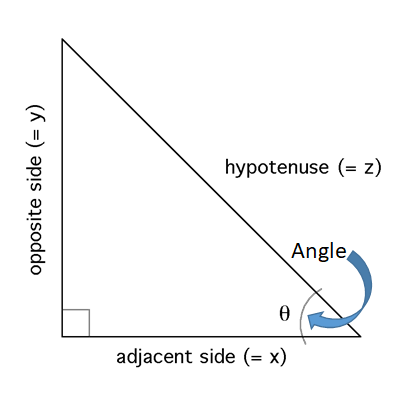tangent of an angle is the angle made by the side and hypotenuse Tangent (Angle()) = opposite site ( = y ) / adjacent site ( = x )
TAN () = x / z

So, it means tangent function returns the ratio of the sides when the angle is given as input. Now if we wish to return the angle when ratio of the sides is given as input, we’ll use the inverse of the tangent function which is the ATAN function. The above explained representation is shown in below equations

= TAN -1( x / z ) { in mathematics }
= ATAN ( x / z ) { in Excel }

This above stated method is used when we need to find the angle of the triangle having given the length of sides. But ATAN2 function returns the angle made by the line connecting the coordinates ( x , y ) to origin with x - axis.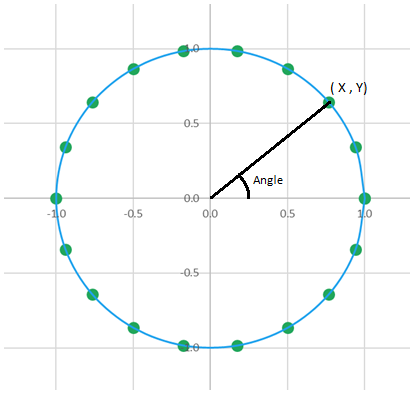ATAN2 function in excel returns the angle value in radians, the function takes the two coordinates of the point ( x_num, y_num)
Syntax:

= ATAN2 ( x_num, y_num )

x_num : The x-coordinate of the point.
y_num : The y-coordinate of the point.
Let’s understand more about the ATAN2 function using it in an example: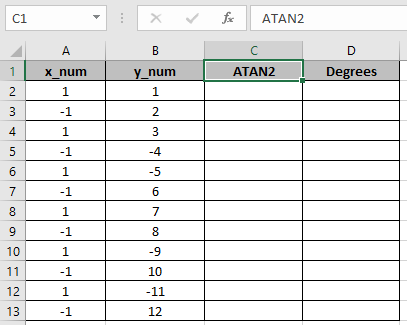Here we have the coordinates of the points in the Cartesian coordinate system having x_num denoting x coordinate and y_num denoting y coordinate. So, we need to find the angle made by the line connecting the coordinates ( x , y ) to origin with x - axis using the ATAN2 function.

Use the formula:

=ATAN2( A2, B2 )

A2 : x_num argument
B2 : y_num argument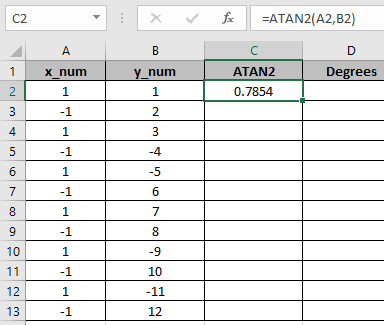Here the argument to the function is given as cell reference. Copy the formula to other cells using the drag down option in excel.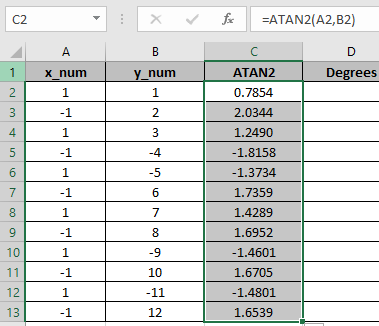As you can see, the ATAN2 function returns a value in radians.

The above method returns the value in radians. So to convert the value from radians to degrees use the DEGREES function or multiply the result with 180/PI().

Use the formula:

= DEGREES ( ATAN2 ( A2, B2 )
OR
= TAN2 ( A2, B2 ) * 180 / PI()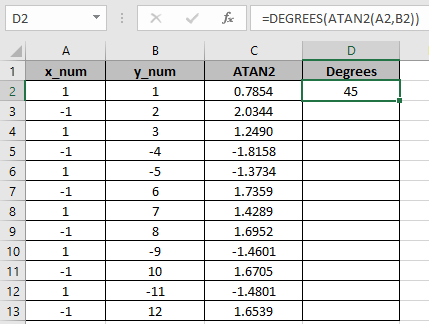The above snapshot gets the result as 45 degrees.
Copy the formula to other cells using the drag down option in excel.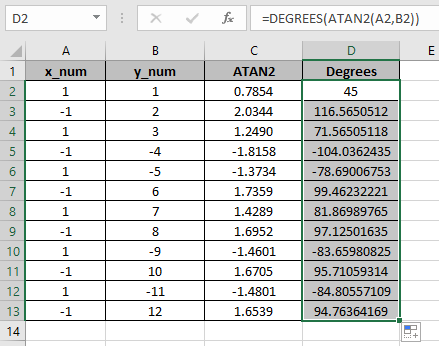The above snapshot use the DEGREES function which takes the input in radians and returns the value in degrees.

Here are some of the observations gathered using the ATAN function.

Note:

1. The function returns in the range of -1.57.. ( -90 degrees ) to +1.57.. ( 90 degrees ).
2. The function returns #VALUE! Error if the argument to the function is non numeric.
3. Use the ATAN function if you need to find the angle having sides of triangle.
4. If the both x_num & y_num argument is zero, the function returns #DIV! Error.
5. ATAN2( a, b) function is same as the ATAN( b / a), but in ATAN2 function the a value can take 0 as value.
6. Positive outcome of the function represents the counterclockwise angle from the x-axis and negative outcome represents the clockwise angle.

As you can see, we can get the inverse of the tangent function using ATAN function.

Hope you understood How to use ATAN function in Excel. Explore more articles on Mathematical function like ASIN function and ACOS function here. Please state your query in the comment box below.

Related Articles

How to use the TANH function in Excel

How to use the TAN function in Excel

How to use Wildcards in Excel

Popular Articles

Edit a dropdown list

If with conditional formatting

If with wildcards

Vlookup by date

Terms and Conditions of use

The applications/code on this site are distributed as is and without warranties or liability. In no event shall the owner of the copyrights, or the authors of the applications/code be liable for any loss of profit, any problems or any damage resulting from the use or evaluation of the applications/code.